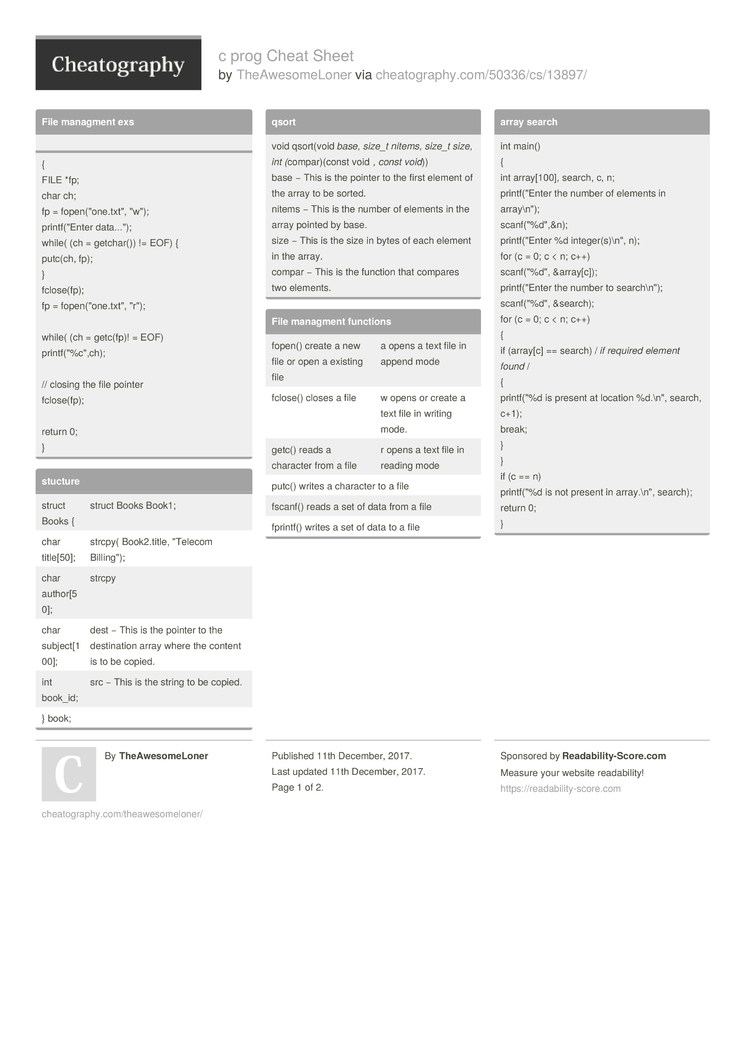Cheatography

# c prog Cheat Sheet by TheAwesomeLoner

### File managment exs

{
FILE *fp;
char ch;
fp = fopen(­"­one.tx­t", "­w");
printf­("Enter data...");
while( (ch = getchar()) != EOF) {
putc(ch, fp);
}
fclose­(fp);
fp = fopen(­"­one.tx­t", "­r");

while( (ch = getc(fp)! = EOF)
printf­("%c­"­,ch);

// closing the file pointer
fclose­(fp);

return 0;
}

### stucture

 struct Books { struct Books Book1; char title; strcpy( Book2.t­itle, "­Telecom Billin­g"); char author­; strcpy char subjec­t; dest − This is the pointer to the destin­ation array where the content is to be copied. int book_id; src − This is the string to be copied. } book;

### qsort

 void qsort(void base, size_t nitems, size_t size, int (comp­ar)­(const void , const void)) base − This is the pointer to the first element of the array to be sorted. nitems − This is the number of elements in the array pointed by base. size − This is the size in bytes of each element in the array. compar − This is the function that compares two elements.

### File managment functions

 fopen() create a new file or open a existing file a opens a text file in append mode fclose() closes a file w opens or create a text file in writing mode. getc() reads a character from a file r opens a text file in reading mode putc() writes a character to a file fscanf() reads a set of data from a file fprintf() writes a set of data to a file

### array search

 int main() { int array[­100], search, c, n; printf­("Enter the number of elements in array­\n"); scanf(­"­%d",­&n); printf­("Enter %d intege­r(s­)\n­", n); for (c = 0; c < n; c++) scanf(­"­%d", &a­rra­y[c]); printf­("Enter the number to search­\n"); scanf(­"­%d", &s­earch); for (c = 0; c < n; c++) { if (array[c] == search) / if required element found / { printf­("%d is present at location %d.\n", search, c+1); break; } } if (c == n) printf­("%d is not present in array.­\n", search); return 0; }

### c - bits manipu­lation

 & Binary AND Operator copies a bit to the result if it exists in both operands. (A & B) will give 12 which is 0000 1100 | Binary OR Operator copies a bit if it exists in eather operand. (A | B) will give 61 which is 0011 1101 ^ Binary XOR Operator copies the bit if it is set in one operand but not both. (A ^ B) will give 49 which is 0011 0001 ~ Binary Ones Complement Operator is unary and has the efect of 'flipping' bits. (~A ) will give -60 which is 1100 0011 << Binary Left Shift Operator. The left operands value is moved left by the number of bits specified by the right operand. A << 2 will give 240 which is 1111 0000

### char array

 char p[] = "­hel­lo" char *p = "­hel­lo"2 Pages
//media.cheatography.com/storage/thumb/theawesomeloner_c-prog.750.jpg

PDF (recommended)